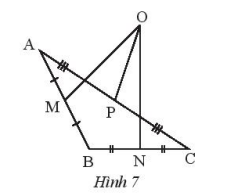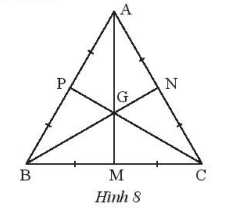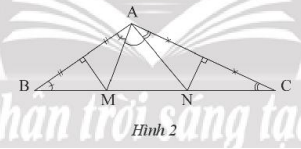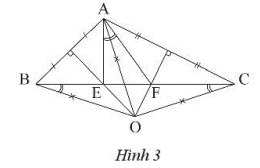## Solving SBT Lesson 6: Properties of three perpendicular bisectors of a triangle (C8 SBT Math 7 Horizons) – Math Book

SBT Solution Lesson 6: Properties of the three perpendicular bisectors of a triangle (C8 SBT Math 7 Horizons)
==========

### Solve problem 1 page 57 SBT Math 7 Creative horizon episode 2 – CTST

Is point O in figure 7 the intersection of the three perpendicular bisectors of triangle ABC? Please explain.Detailed instructions for solving Lesson 1

Solution method

Prove that OM is not perpendicular to AB

Detailed explanation

Since OM is not perpendicular to AB, O is not the intersection of the three perpendicular bisectors of triangle ABC.

–>

— *****

### Solve problem 2 page 57 SBT Math 7 Creative horizon episode 2 – CTST

Given an equilateral triangle ABC and a point G as shown in Figure 8. Prove GA = GB = GC.Detailed instructions for solving Lesson 2

Solution method

Prove that G is the intersection of the perpendicular bisector of triangle ABC.

Detailed explanation

We have: AB = AC, MA = MC, so AM is the midpoint of BC.

Similarly we also have BN is the perpendicular bisector of AC, CP is the perpendicular bisector of AB.

Point G is the intersection of the perpendicular bisector of triangle ABC, so we have GA = GB = GC

–>

— *****

### Solve problem 3 page 58 SBT Math 7 Creative horizon episode 2 – CTST

Let ABC be a triangle with angle A equal to $${120^o}$$. The perpendicular bisectors of AB and Ac intersect Bc at M and N respectively. Calculate the measure of angle MAN.

Detailed instructions for solving Lesson 3

Solution method

Using property of isosceles triangle

Detailed explanationWe have MA = MB deduce triangle MAB isosceles at M deduce $$\widehat {MAB} = \widehat {MBA} = \widehat B$$

Similarly, we have an isosceles triangle NAC at N so $$\widehat {NAC} = \widehat {NCA} = \widehat C$$

We have: $$\widehat {MAN} = \widehat {BAC} – \left( {\widehat {MAB} + \widehat {NAC}} \right) = {120^o} – \left( {\widehat B + \widehat C} \right) = {120^o} – {60^o} = {60^o}$$

–>

— *****

### Solve problem 4 page 58 SBT Math 7 Creative horizon episode 2 – CTST

Let ABC be a triangle with angle A being the magnetic angle. The perpendicular bisectors of AB and Ac intersect at O ​​and intersect BC at E and F respectively. Prove

a) $$\Delta EO{\rm{A}} = \Delta EOB,\Delta F{\rm{O}}A = \Delta F{\rm{O}}C$$

b) Prove that AO is the bisector of angle EAF.

Detailed instructions for solving Lesson 4

Solution method

Find the conditions for two triangles to be congruent.

– Prove: $$\widehat {OA{\rm{E}}} = \widehat {OAF}$$ infer that AO is the bisector of angle EAF

Detailed explanationa) E and O are on the perpendicular bisector of AB, so we have EA = EB, OA = OB.

F and O lie on the perpendicular bisector of AC, so we have FA = FC, OA = OC

Infer: $$\Delta EO{\rm{A}} = \Delta EOB$$(c – c – c) because of common EO, EA = EB, OA = OB

$$\Delta F{\rm{O}}A = \Delta F{\rm{O}}C(c – c – c)$$ for common FO, FA = FC, OA = OC.

b) We have OA = OC and OA = OB so OB = OC deduce triangle OBC isosceles at O ​​deduce $$\widehat {OBE} = \widehat {OCF}(1)$$

We have $$\Delta EO{\rm{A}} = \Delta EOB$$; $$\Delta F{\rm{O}}A = \Delta F{\rm{O}}C$$ hence: $$\widehat {OA{\rm{E}}} = \widehat {OBE} ;\widehat {OAF} = \widehat {OCF}(2)$$

From (1) and (2) we have: $$\widehat {OA{\rm{E}}} = \widehat {OAF}$$ infer that AO is the bisector of angle EAF.

–>

— *****

### Solve problem 5 page 58 SBT Math 7 Creative horizon episode 2 – CTST

Let ABC be a triangle with a median perpendicular to AC passing through vertex B, prove triangle ABC is an isosceles triangle

Detailed instructions for solving Lesson 5

Solution method

Prove that BA = BC infers an isosceles triangle ABC.

Detailed explanation

Point B lies on the perpendicular bisector of AC, so BA = BC. It follows that triangle ABC is an isosceles triangle at B.

–>

— *****